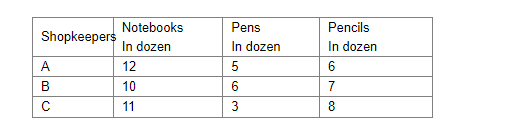# Three shopkeepers A, B and C go to a store to buy stationary. A purchases 12 dozen notebooks,

Question:

Three shopkeepers AB and C go to a store to buy stationary. A purchases 12 dozen notebooks, 5 dozen pens and 6 dozen pencils. B purchases 10 dozen notebooks, 6 dozen pens and 7 dozen pencils. C purchases 11 dozen notebooks, 13 dozen pens and 8 dozen pencils. A notebook costs 40 paise, a pen costs Rs. 1.25 and a pencil costs 35 paise. Use matrix multiplication to calculate each individual's bill.

Solution:Here,

Cost of notebooks per dozen $=(12 \times 40)$ paise $=$ Rs $4.80$

Cost of pens per dozen = Rs. $(12 \times 1.25)=$ Rs 15

Cost ofpPencils per dozen $=(12 \times 35)$ paise $=$ Rs $4.20$

$\therefore\left[\begin{array}{ccc}12 & 5 & 6 \\ 10 & 6 & 7 \\ 11 & 13 & 8\end{array}\right]\left[\begin{array}{c}4.80 \\ 15 \\ 4.20\end{array}\right]=\left[\begin{array}{c}12 \times 4.80+5 \times 15+6 \times 4.20 \\ 10 \times 4.80+6 \times 15+7 \times 4.20 \\ 11 \times 4.80+13 \times 15+8 \times 4.20\end{array}\right]$

$=\left[\begin{array}{c}57.60+75+25.20 \\ 48+90+29.40 \\ 52.80+195+33.60\end{array}\right]$

$=\left[\begin{array}{l}157.80 \\ 167.40 \\ 281.40\end{array}\right]$

Thus, the bills of A, B and C are Rs $157.80$, Rs $167.40$ and Rs $281.40$, respectively.